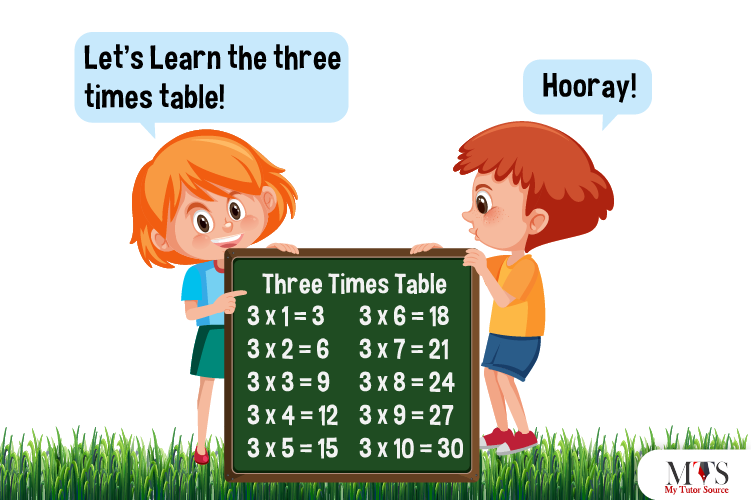# Multiplication Table of 3 – Tips to Memorize 3 Times Table & Example QuestionsAfter memorizing the table of 2 on fingertips, the multiplication table of 3 would be less of a daunting task. Math comes with tricky and so its multiplication tables. Here we have mentioned a few tricks and fun learning games to help the kids memorize the table of 3 faster and quicker than any other table. Tips of learning are designed after considering the potential of personalized learning. Our private tutors follow the same multiplication learning strategies.

## Table of 3

Repeated addition of 3 makes the table of three, for instance, 3 + 3 + 3 = 9, which is equivalent to 3 x 3. Memorizing times tables is a kind of brain training activity started by traditional teaching methodologies to develop and sharpen the arithmetic skills of the students. It’s one of the essential skills helpful in the long run.

Below we have given the table of 3 up to 20; give it a read.

3 x 1 = 3
3 x 2 = 6
3 x 3 = 9
3 x 4 = 12
3 x 5 = 15
3 x 6 = 18
3 x 7 = 21
3 x 8 = 24
3 x 9 = 27
3 x 10 = 30
3 x 11 = 33
3 x 12 = 36
3 x 13 = 39
3 x 14 = 42
3 x 15 = 45
3 x 16 = 48
3 x 17 = 51
3 x 18 = 54
3 x 19 = 57
3 x 20 = 60

## Method to Read Table of 3

This is how we read the multiplication table of 3 for learning purposes:

One time three is three (3)
Two times three is six (6)
Three times three is nine (9)
Four times three is twelve (12)
Five times three is fifteen (15
Six times three is eighteen (18)
Seven times three is twenty-one (21)
Eight times three is twenty-four (24)
Nine times three is twenty-seven (27)
Ten times three is thirty (30)
Eleven times three is thirty-three (33)
Twelve times three is thirty-six (36)
Thirteen times three is thirty-nine (39)
Fourteen times three is forty-two (42)
Fifteen times three is forty-five (45)
Sixteen times three is forty-eight (48)
Three times three is fifty-one (51)
Eighteen times three is fifty-four (54)
Nineteen times three is fifty-seven (57)
Twenty times three is sixty (60)

## Tips for Memorizing Multiplication Table of 3

There are always numerous ways to read, learn and memorize tasks. That’s the same case with the multiplication table of 3. picking the right teaching strategy is the primary part because wrong learning theories always discourage kids and slow down the learning process. Here are a few tips to follow for memorizing 3 times table:

### 1. Read, Practice and Repeat

Read, recite, repeat with complete attention. Mastering a skill, one must practice a lot. Memorizing tables of mathematics demand the same. Students must read loudly and repeat until it is on their fingertips.

Number one rule to master multiplication is using your addition skills as multiplication is all about repeated addition, nothing else. Let us take an example using a 3 times table: to find the answer of 3 x 5, all you have to do is add 3 five-time, and it is equivalent to 3 x 5 products. This is how,

• Product of 3 x 5 = 15
• Addition of 3 + 3 + 3 + 3 + 3 = 15

### 3. Matching Games

Students perform better when taught using fun activities and games. They must play a matching game of 3 tables. It is one of the most exciting and engaging tips to memorize multiplication tables.

Learn 3 times table with a pattern. It follows the same pattern for every ten multiples, such as 3, 6, 9, 12, 14, 18, 21, 24, 27, 30. There’s no other rule for quick learning.

### 5. Short Quizzes and Questions

Another exciting tip for testing the knowledge of students and helping them with learning is using multiple-choice questions, surprise quizzes, and fill-in-the-blank spaces’ methods.

## Example Question of Table of 3

Fill in the blanks using a multiplication table of 3 times. For example: 3 x 2 = 6

3 x 7 = ___

3 x ___ = 12

___ x 19 = 57

3 x ___ = 39

___ x ___ = 33

3 x 9 = ___

3 x ___ = 18

___ x ___ = 60

3 x 14 = ___

Find the value of 5 plus 3 times 8?

Solution:

Given that,
5 plus 3 times 8
In mathematical form
5 + 3 x 8 = 5 + 24 = 29
Hence, 5 plus 3 times 8 is equal to 29.

Pick the multiples of 3 from the following table.

The multiples of 3 times table in the given table are:
3, 6, 9, 12, 15, 18, 21, 24, 27, 30, 33, 36, 39 and 42

Tony reads 3 pages of a book daily. How many pages will he read in 20 days?

Solution:

To find the total number of pages within 20 days, we will
3 x 20 = 60
Hence, Tony will read 60 pages in 20 days

How many threes are in 27?

Using multiplication table of 3
3 x 9 = 27
Hence, there are 9 threes in 27.

### Find Top Tutors in Your AreaWith over 3 years of experience in teaching, Chloe is very deeply connected with the topics that talk about the educational and general aspects of a student's life. Her writing has been very helpful for students to gain a better understanding of their academics and personal well-being. I’m also open to any suggestions that you might have! Please reach out to me at chloedaniel402 [at] gmail.com